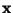Next: Principal component analysis Up: Classical Linear Transformations Previous: Introduction

## Second-order methods

The most popular methods for finding a linear transform as in Eq. (2) are second-order methods. This means methods that find the representation using only the information contained in the covariance matrix of the data vector. Of course, the mean is also used in the initial centering. The use of second-order techniques is to be understood in the context of the classical assumption of Gaussianity. If the variablehas a normal, or Gaussian distribution, its distribution is completely determined by this second-order information. Thus it is useless to include any other information. Another reason for the popularity of the second-order methods is that they are computationally simple, often requiring only classical matrix manipulations.

The two classical second-order methods are principal component analysis and factor analysis, see [51,77,87]. One might roughly characterize the second-order methods by saying that their purpose is to find a faithful representation of the data, in the sense of reconstruction (mean-square) error. This is in contrast to most higher-order methods (see next Section) which try to find a meaningful representation. Of course, meaningfulness is a task-dependent property, but these higher-order methods seem to be able to find meaningful representations in a wide variety of applications [36,46,80,81].Next: Principal component analysis Up: Classical Linear Transformations Previous: Introduction
Aapo Hyvarinen
1999-04-23